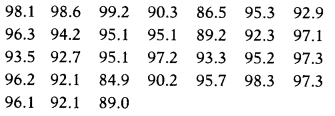# The relative humidity (in %) of a certain city for a month of 31 days was as follows

The relative humidity (in %) of a certain city for a month of 31 days was as followsFind the probability of relative humidity less than 94% on any of these days.

Given, total number of days = 31
∴Total number of trials = 31
Let E be the event of getting a day when the relative humidity less than 94%.
∴ Number of trials in which E happened = 13